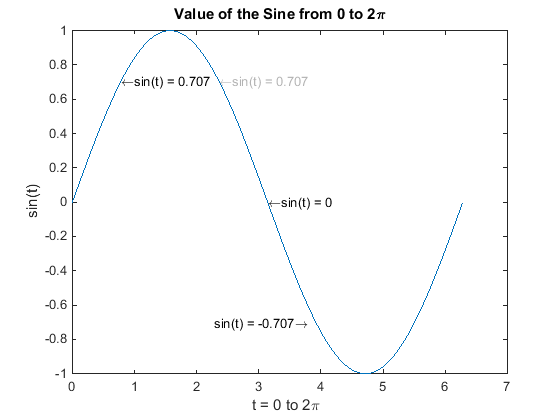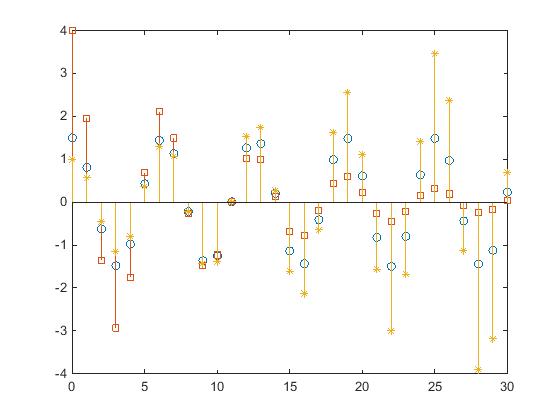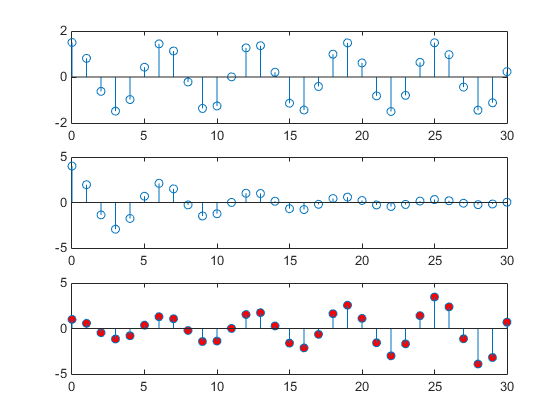## Find Objects

### Find Objects with Specific Property Values

The `findobj` function can scan the object hierarchy to obtain the handles of objects that have specific property values.

For identification, all graphics objects have a `Tag` property that you can set to any character vector. You can then search for the specific property/value pair. For example, suppose that you create a check box that is sometimes inactivated in the UI. By assigning a unique value for the `Tag` property, you can find that particular object:

`uicontrol('Style','checkbox','Tag','save option')`

Use `findobj` to locate the object whose `Tag` property is set to `'save option`' and disable it:

```hCheckbox = findobj('Tag','save option'); hCheckbox.Enable = 'off'```

If you do not specify a starting object, `findobj` searches from the root object, finding all occurrences of the property name/property value combination that you specify.

To find objects with hidden handles, use `findall`.

### Find Text by String Property

This example shows how to find text objects using the `String` property.

The following graph contains text objects labeling particular values of the function.Suppose that you want to move the text labeling the value sin(t) = .707 from its current location at `[pi/4,sin(pi/4)]` to the point `[3*pi/4,sin(3*pi/4)]` where the function has the same value (shown in light gray out in the graph).

Determine the handle of the text object labeling the point `[pi/4,sin(pi/4)]` and change its `Position` property.

To use `findobj`, pick a property value that uniquely identifies the object. This example uses the text `String` property:

`hText = findobj('String','\leftarrowsin(t) = .707');`

Move the object to the new position, defining the text `Position` in axes units.

`hText.Position = [3*pi/4,sin(3*pi/4),0];`

`findobj` lets you restrict the search by specifying a starting point in the hierarchy, instead of beginning with the root object. If there are many objects in the object tree, this capability results in faster searches. In the previous example, you know that the text object of interest is in the current axes, so you can type:

`hText = findobj(gca,'String','\leftarrowsin(t) = .707');`

### Use Regular Expressions with findobj

This example shows how to find object handles using regular expressions to identify specific property values. For more information about regular expressions, see `regexp`.

Suppose that you create the following graph and want to modify certain properties of the objects created.

```x = 0:30; y = [1.5*cos(x);4*exp(-.1*x).*cos(x);exp(.05*x).*cos(x)]'; h = stem(x,y); h(1).Marker = 'o'; h(1).Tag = 'Decaying Exponential'; h(2).Marker = 'square'; h(2).Tag = 'Growing Exponential'; h(3).Marker = '*'; h(3).Tag = 'Steady State';```Passing a regular expression to `findobj` enables you to match specific patterns. For example, suppose that you want to set the value of the `MarkerFaceColor` property to green on all stem objects that do not have their `Tag` property set to `'Steady State'` (that is, stems that represent decaying and growing exponentials).

```hStems = findobj('-regexp','Tag','^(?!Steady State\$).'); for k = 1:length(hStems) hStems(k).MarkerFaceColor = 'green' end```

### Limit Scope of Search

Specify the starting point in the object tree to limit the scope of the search. The starting point can be the handle of a figure, axes, or a group of object handles.

For example, suppose that you want to change the marker face color of the stems in a specific axes:

```x = 0:30; y = [1.5*cos(x);4*exp(-.1*x).*cos(x);exp(.05*x).*cos(x)]'; tiledlayout(3,1) ax1 = nexttile; stem(x,y(:,1)) ax2 = nexttile; stem(x,y(:,2)) ax3 = nexttile; stem(x,y(:,3))```

Set the marker face color of the stems in the third axes only.

```h = findobj(ax3,'Type','stem'); h.MarkerFaceColor = 'red';```For more information on limiting the scope and depth of an object search, see `findobj` and `findall`.